# 因為LRU設計效率太低被pass了

### 前言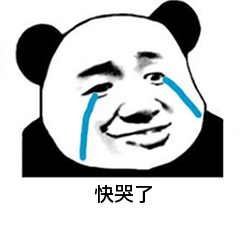### 理解LRU

LRU，英文全稱為Least Recently Used，翻譯過來就是最近最久未使用演算法，是一種常用的頁面置換演算法

LRU理解不難，就是維護一個有固定大小的容器，核心就是get()和put()兩個操作。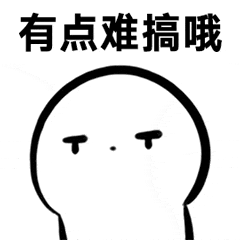```容量大小為2： [ "put", "put", "get", "put","get", "put","get","get","get"] [ [1, 1], [2, 2], , [3, 3], , [4, 4], , , ]```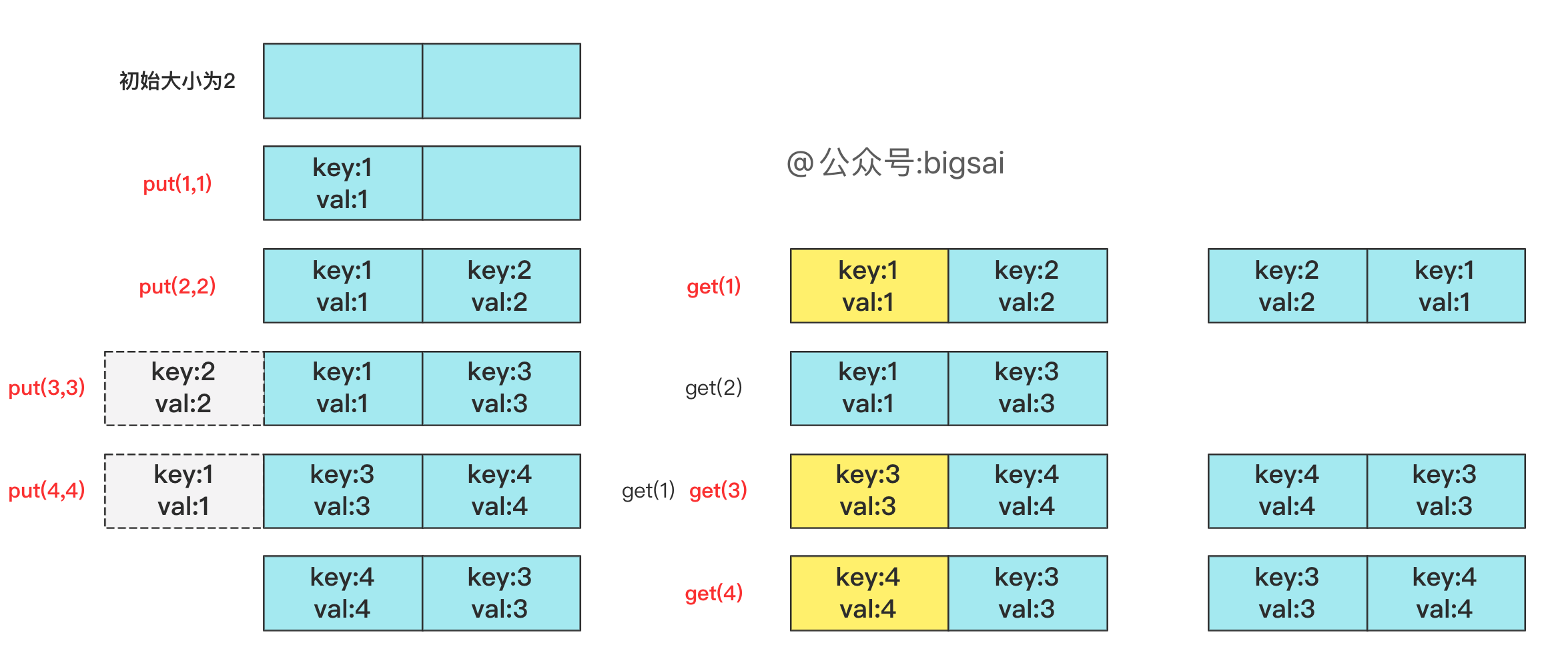• put()存在更新的操作,例如put(3,3),put(3,4)會更新key為3的操作。
• get()可能查詢不到，但是查詢到也會更新最久未使用的順序
• 如果容器未使用滿，那麼put可能更新可能插入，但是不會刪除；如果容器滿了並且put插入，就要考慮刪除最久未使用的key-value了。

put操作：O(n)，get操作：O(n) 兩個操作都需要列舉列表線性複雜度，效率屬實有點拉胯，肯定不行，這樣的程式碼我就不寫了。### 雜湊初優化

```java class LRUCache {

``````Map<Integer,Integer>map=new HashMap<>();
List<Integer>list=new ArrayList<>();
int maxSize;
public  LRUCache(int capacity) {
maxSize=capacity;
}

public int get(int key) {
if(!map.containsKey(key))//不存在返回-1
return -1;
int val=map.get(key);
put(key,val);//要更新位置 變成最新 很重要！
return val;
}

public void put(int key, int value) {
//如果key存在，直接更新即可
if (map.containsKey(key)) {
list.remove((Integer) key);
} else {//如果不存在 要插入到最後，但是如果容量滿了需要刪除第一個(最久)
if (!map.containsKey(key)) {
if (list.size() == maxSize) {
map.remove(list.get(0));
list.remove(0);
}
}
}
map.put(key, value);
}
``````

} ```

### 雜湊+雙鏈表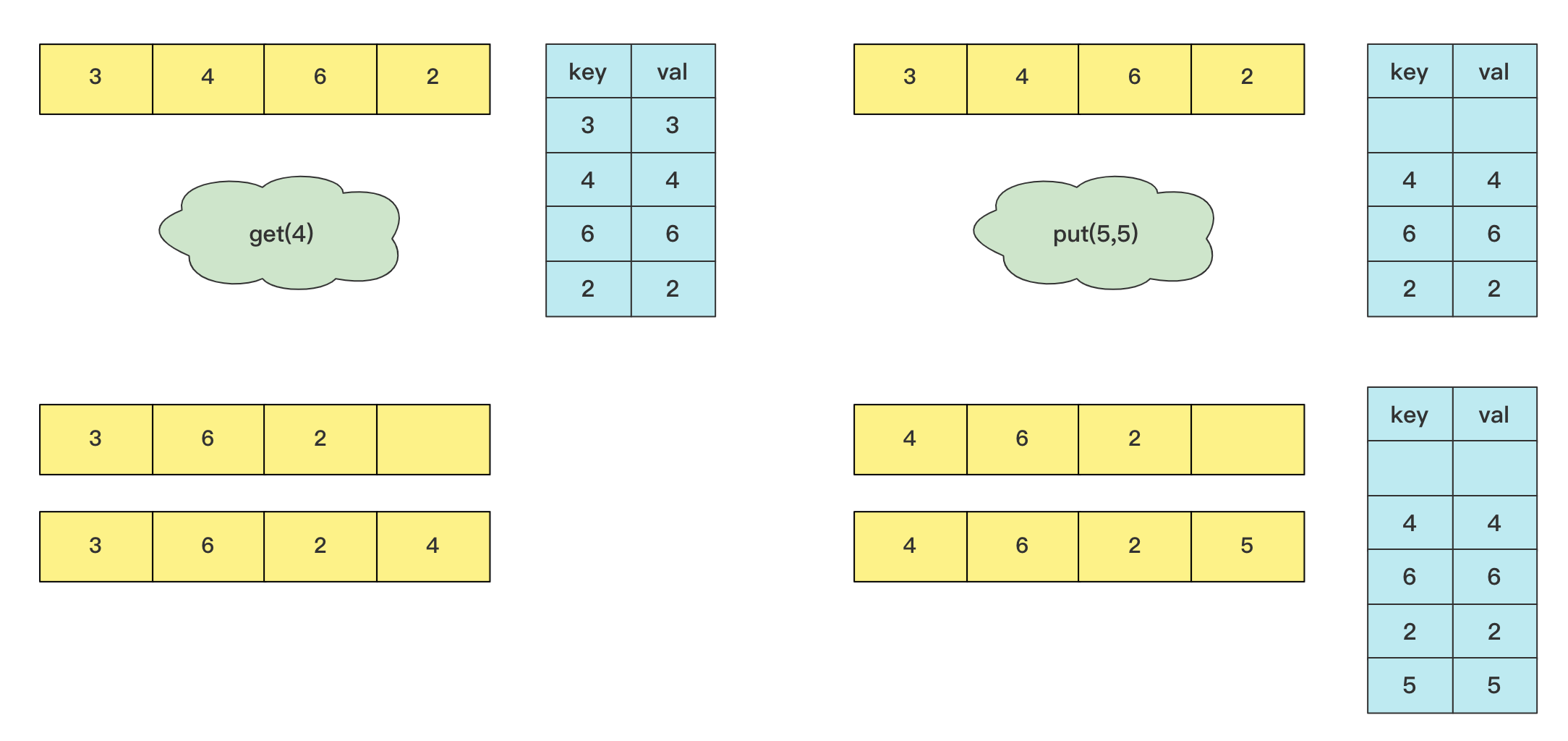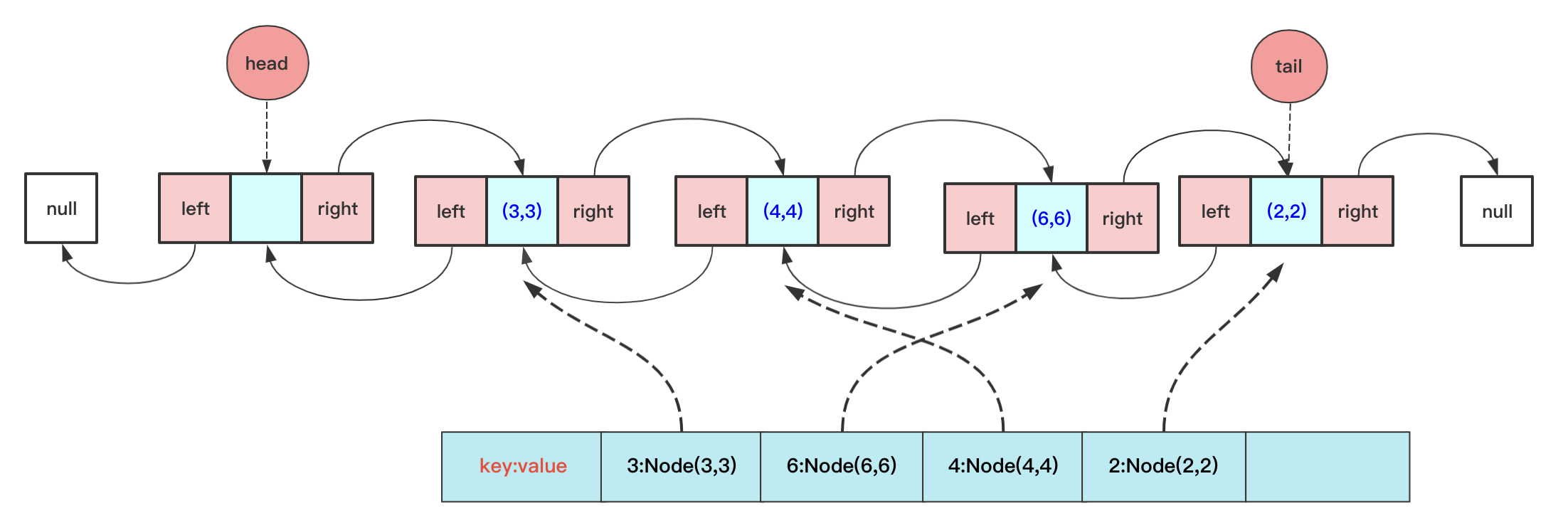```java class LRUCache { class Node { int key; int value; Node pre; Node next;

``````    public Node() {
}

public Node( int key,int value) {
this.key = key;
this.value=value;
}
}
class DoubleList{
private Node tail;// 尾節點
private int length;
public DoubleList() {
length = 0;
}
{
tail.next = teamNode;
teamNode.pre=tail;
tail = teamNode;
length++;
}
void deleteFirst(){
return;

length--;
}
void deleteNode(Node team){

team.pre.next=team.next;
if(team.next!=null)
team.next.pre=team.pre;
if(team==tail)
tail=tail.pre;
team.pre=null;
team.next=null;
length--;
}
public String toString() {
String vaString = "len:"+length+" ";
while (team != null) {
vaString +="key:"+team.key+" val:"+ team.value + " ";
team = team.next;
}
return vaString;
}
}
Map<Integer,Node> map=new HashMap<>();
DoubleList doubleList;//儲存順序
int maxSize;

public   LRUCache(int capacity) {
doubleList=new DoubleList();
maxSize=capacity;
}
public  void print(){
System.out.print("maplen:"+map.keySet().size()+" ");
for(Integer in:map.keySet()){
System.out.print("key:"+in+" val:"+map.get(in).value+" ");
}
System.out.print("              ");
System.out.println("listLen:"+doubleList.length+" "+doubleList.toString()+" maxSize:"+maxSize);
}

public int get(int key) {
int val;
if(!map.containsKey(key))
return  -1;
val=map.get(key).value;
Node team=map.get(key);
doubleList.deleteNode(team);
return  val;
}

public void put(int key, int value) {
if(map.containsKey(key)){// 已經有這個key 不考慮長短直接刪除然後更新
Node deleteNode=map.get(key);
doubleList.deleteNode(deleteNode);
}
else if(doubleList.length==maxSize){//不包含並且長度小於
map.remove(first.key);
doubleList.deleteFirst();
}
Node node=new Node(key,value);
map.put(key,node);

}
``````

}

```

### 尾聲

```java class LRUCache extends LinkedHashMap{ private int capacity;

``````public LRUCache(int capacity) {
super(capacity, 0.75F, true);
this.capacity = capacity;
}

public int get(int key) {
return super.getOrDefault(key, -1);
}

public void put(int key, int value) {
super.put(key, value);
}

@Override
protected boolean removeEldestEntry(Map.Entry<Integer, Integer> eldest) {
return size() > capacity;
}
``````

} ```

「其他文章」